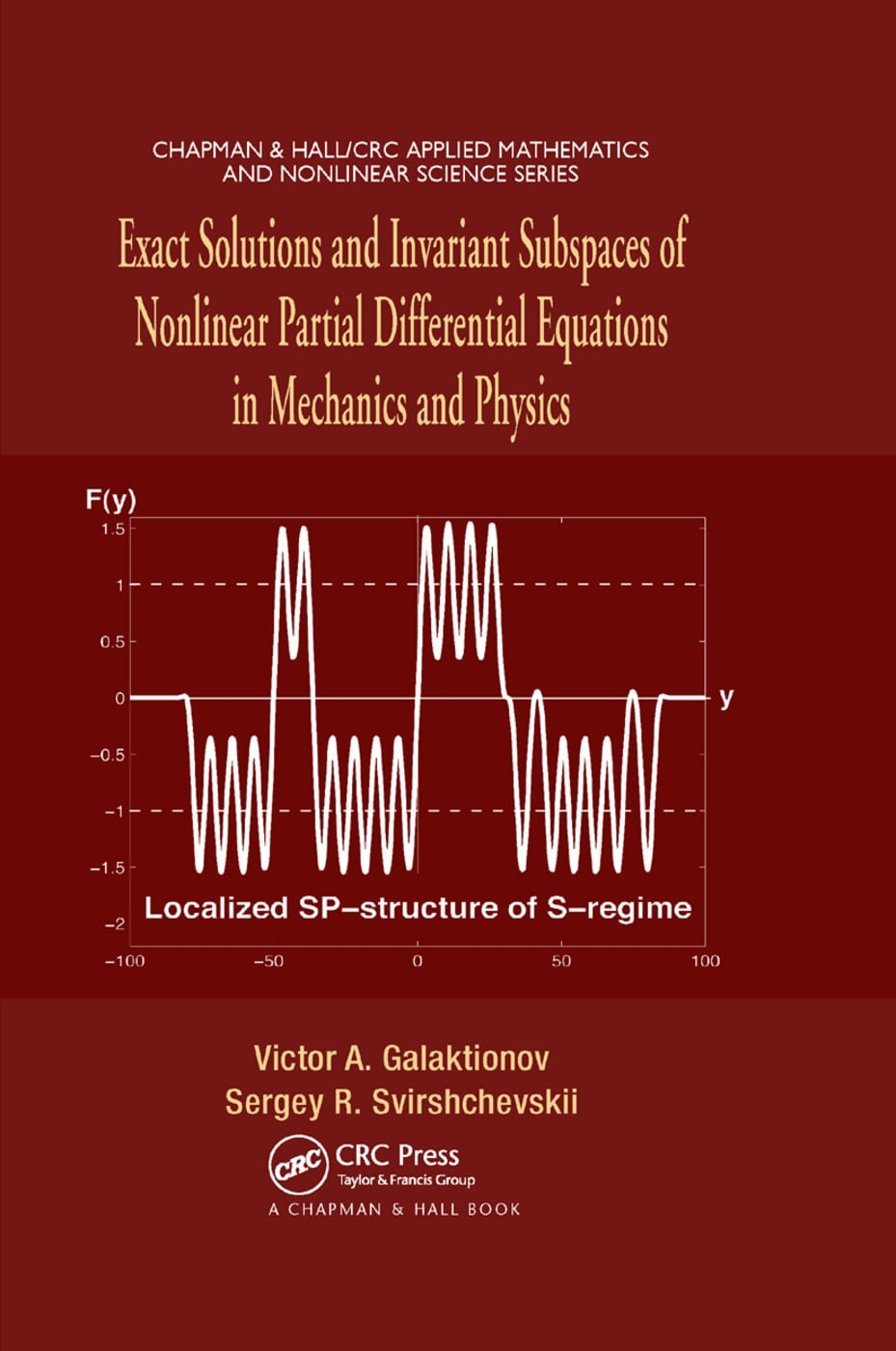# Exact Solutions and Invariant Subspaces of Nonlinear Partial Differential Equations in Mechanics and Physics

## 1st Edition

Chapman and Hall/CRC

528 pages

Paperback: 9780367389970
pub: 2019-09-19
SAVE ~\$14.99
\$74.95
\$59.96
x
Hardback: 9781584886631
pub: 2006-11-02
SAVE ~\$28.00
\$140.00
\$112.00
x
eBook (VitalSource) : 9780429149306
pub: 2006-11-02
from \$28.98

FREE Standard Shipping!

### Description

Exact Solutions and Invariant Subspaces of Nonlinear Partial Differential Equations in Mechanics and Physics is the first book to provide a systematic construction of exact solutions via linear invariant subspaces for nonlinear differential operators. Acting as a guide to nonlinear evolution equations and models from physics and mechanics, the book focuses on the existence of new exact solutions on linear invariant subspaces for nonlinear operators and their crucial new properties.

This practical reference deals with various partial differential equations (PDEs) and models that exhibit some common nonlinear invariant features. It begins with classical as well as more recent examples of solutions on invariant subspaces. In the remainder of the book, the authors develop several techniques for constructing exact solutions of various nonlinear PDEs, including reaction-diffusion and gas dynamics models, thin-film and Kuramoto-Sivashinsky equations, nonlinear dispersion (compacton) equations, KdV-type and Harry Dym models, quasilinear magma equations, and Green-Naghdi equations. Using exact solutions, they describe the evolution properties of blow-up or extinction phenomena, finite interface propagation, and the oscillatory, changing sign behavior of weak solutions near interfaces for nonlinear PDEs of various types and orders.

The techniques surveyed in Exact Solutions and Invariant Subspaces of Nonlinear Partial Differential Equations in Mechanics and Physics serve as a preliminary introduction to the general theory of nonlinear evolution PDEs of different orders and types.

Introduction. Linear Invariant Subspaces in Quasilinear Equations. Invariant Subspaces and Modules. Parabolic Equations in One Dimension. Odd-Order One-Dimensional Equations. Quasilinear Wave and Boussinesq Models in One Dimension. Applications to Partial Differential Equations in IRN. Partially Invariant Subspaces, Invariant Sets, and Generalized Separation of Variables. Sign-Invariants for Second-Order Parabolic Equations and Exact Solutions. Invariant Subspaces for Discrete Operators, Moving Mesh Methods, and Lattices. References. List of Frequently Used Abbreviations. Index.

Galaktionov, Victor A.; Svirshchevskii, Sergey R.